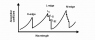vertices
Probably a stupid question but when the energy of x ray photons striking atomic electrons increase, why is it that the probability of photoionisation decreases?

I mean, obviously there are absorption edges corresponding to the K, L, M etc edges but the *general* trend is that the the absorption probability decreases with increasing photon energy. Why is this the case?

wasia
I cannon answer clearly, but some remarks may be relevant:
1) Photoionisation is a process of resonant nature ( maybe that's what you meant )
2) Higher energy means that the photon has to penetrate to deeper electronic levels to
3) Other mechanisms such as scattering are important

vertices
I cannon answer clearly, but some remarks may be relevant:
1) Photoionisation is a process of resonant nature ( maybe that's what you meant )
2) Higher energy means that the photon has to penetrate to deeper electronic levels to
3) Other mechanisms such as scattering are important

well I'm not really talking about the resonant nature of photoionisation. If you look at the attached file, it shows what I am trying to get across: as the energy of the incoming photon increases (corresponding to a decrease in wavelength), the absorption coefficient goes down. Why is this so?

Yes, at higher energies the photon does have energies to knock out inner electron that are closer to the nucleus but why does the probability that this will happen decrease?

Scattering - because electrons are so light the recoil rather than scatter. In the case of bound electrons, they photoionise. I am not if scattering is relavent here.

Staff Emeritus
Is there a file one meant to attach? Or does one have a reference?

wasia
I find no file attached.

My argument goes like this:

Low energy photons are absorbed by outer shell electrons in resonant fashion.

As energy gets higher, 1) Compton scattering becomes important and 2) resonant absorbtion does not occur at the outskirts of atom.

Compton scattering means that if a photon and electron interact, electron is not freed and photon changes its direction. The change of direction may be NOT towards nucleus - then no photoionisation at all occurs.

Wikipedia entries Photoelectric_effect and Photoionisation_cross_section may be of interest.

vertices

BUT hmmm, I'm getting a little bit confused. I thought compton scattering by definition is inelastic in the sense that it transfers momentum to the electron, making it free (from its bound state).

Sorry for forgetting to attach the file. I do so now.

#### Attachments

•Absorption.png
3.5 KB · Views: 365
Staff Emeritus
Homework Helper
From what I can remember, it's a matter of quantum mechanics and interference.

The transition probability has a term

$$\int \psi_{bound}(x) \ x \ \psi*_{free}(x)d^3x$$

The "bound" state is the electron's particular orbital in the atom.
The "free" state is just a traveling electron wavepacket, exp(ikx) or exp(-ikx).

For high energy photons, the free state has larger kinetic energy, hence larger "k". These short-deBroglie-wavelength states make for a smaller value of the integral represented by the above expression. You can think of it as adjacent half-cycles of exp(ixk) tend to cancel each other out in the integral, if the other terms in the integrand do not change appreciably over one de Broglie wavelength scale.

vertices

One question though:

should:

$$\int \psi_{bound}(x) \ x \ \psi*_{free}(x)d^3x$$

really be:

$$\int \psi_{bound}(r) \ V(r) \ \psi*_{free}(r)d^3r ?$$

as I would think this better expresses the dynamics of the situation?

Staff Emeritus
Homework Helper
I am going from roughly 15-year memory, but as I recall the operator between the two wavefunctions should be the energy of a dipole in an electric field,

$$E \cdot d \$$ (both terms are vectors)

Assuming E is spatially independent, it can be taken outside the integral. Leaving the dipole term d, which for an electron equals -ex, inside the integral. Take out the constant electron charge e, and you're left with the integral I wrote originally, times some physical constants and spatially independent terms.

I'm using x as the position vector, not just the "x-component" of position.

Last edited:
vertices
I am going from roughly 15-year memory, but as I recall the operator between the two wavefunctions should be the energy of a dipole in an electric field,

$$E \cdot d \$$ (both terms are vectors)

Assuming E is spatially independent, it can be taken outside the integral. Leaving the dipole term d, which for an electron equals -ex, inside the integral. Take out the constant electron charge e, and you're left with the integral I wrote originally, times some physical constants and spatially independent terms.